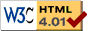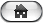Home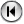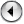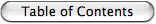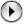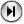First - Prev - Contents - Next - Last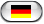Deutsch

# Appendix A: Commodore VIC-20 BASIC V2

Here is a short summary of the commands for the built in Commodore VIC-20 BASIC V2. Note that this is not intended to be a tutorial, but just a quick reference to refresh long unused brain cells.
If you are looking for a BASIC tutorial, take a look at the original VIC-20 manual. It is available as etext at: http://project64.c64.org

In VIC-20 BASIC V2 there are only few types of variables:

• Float: This is the most frequently used type. Float-variables do not carry a type identifier.
Range +/- [2.94E-39 ... 1.70E+38] (+/- [2-128 ... 2127])
Precision: 32 bit mantisse (approx. 9.6 decimal digits) + 8 bit exponent
• Integer (16 Bit): This is a rarely used type. Integer variables carry a '%' at the end of their name (eg. X%). Note that all computations are performed with float precision and conversion to Integer is only performed when the value is assigned to a variable. Thus Integer math is SLOWER(!) than Float math.
• Boolean: Results are expressed using Integers. FALSE is represented by 0 (0x0000) and TRUE by -1 (0xFFFF) or any other non-zero value.
• Strings: String variables carry a '\$' at the end of their name (eg. X\$). Strings can be at most 255 characters long.

Only the first TWO (!) characters of a variable name are significant. Thus 'CO', 'COCOS' and 'COLLOSEUM' (but not 'C' and 'CA') are equivalent names for a single variable. On the other hand the type identifier is used to distinguish variables. Therefore 'CO', 'CO%' and 'CO\$' are three different variables.

## Program Flow Control

`FOR...TO...STEP...NEXT` - The only real loop construct in BASIC
Syntax:
```FOR <Var> = <Start> TO <End> [STEP <Size>] <Loop Code> NEXT [<Var>]```
Examples:
```FOR I=1 TO 5: PRINT I;: NEXT -> 1 2 3 4 5 FOR I=1 TO 5 STEP 2: PRINT I;: NEXT -> 1 3 5 FOR I=5 TO 1 STEP -2: PRINT I;: NEXT I -> 5 3 1 FOR I=3 TO 1: PRINT I;: NEXT -> 3 (!)```
`IF...THEN` - Conditional Program Execution
Note: There is no such thing as `ELSE` or `ENDIF`.
Syntax:
`IF <Condition> THEN <Statements>` or
`IF <Condition> GOTO <LineNr>` or
`IF <Condition> THEN <LineNr>`
Example:
```100 IF A < B THEN MN = A: GOTO 120 110 MN = B 120 ....```
`GOTO` or `GO TO` - Unconditional Jump
Syntax:
`GOTO <LineNr>` or
`GO TO <LineNr>`
`GOSUB` - Unconditional Jump to Subroutine
Note that it is not possible to use formal parameters to a subroutine. Everything must be done using global variables.
Syntax:
`GOSUB <LineNr>`
Example:
```10 PRINT "Main Program" 20 GOSUB 100 30 PRINT "Back To Main" 40 GOSUB 100 50 PRINT "Once again Main" 60 END 100 PRINT "This is the Subroutine" 110 RETURN```
`RETURN` - Return from Subroutine
Syntax:
`RETURN`
Example:
See `GOSUB`
`ON...GOTO` or `ON...GOSUB` - Multiway branch
Syntax:
` ON <IntegerExpr> GOTO <LineNr1>, <LineNr2>` ...
or `ON <IntegerExpr> GOSUB <LineNr1>, <LineNr2>` ...
Example:
`ON X GOTO 100, 200, 300`
This is equivalent to:
```IF X = 1 THEN GOTO 100 IF X = 2 THEN GOTO 200 IF X = 3 THEN GOTO 300```
`DEF FN`- Define a BASIC Function/Subroutine
Syntax:
`DEF FN <Name>(<Param>) = <Single Line Expression>`
Example:
```DEF FN SI(X) = SIN(X)/X FN SI(π/3) -> 0.826993343```

## Input/Output

`GET` - Read One Character from Standard Input without waiting
Syntax:
`GET <VarName>`
Example:
`100 GET A\$: IF A\$ = "" THEN GOTO 100` -> Wait for any Key
`INPUT` - Get Data from Standard Input (usually Keyboard)
Syntax:
`INPUT` `[<Prompt>;] <VarName> [, <VarName> ...]`
Examples:
```INPUT "LOGIN:"; LG\$ INPUT "Please Enter A, B and C"; A, B, C INPUT A```
`PRINT` - Write to Standard Output (usually Screen)
Syntax:
`PRINT <Data>` or
`? <Data>`
Examples:
```PRINT "Hello World" PRINT "Here", "are", "Tabs" -> Note the ',' PRINT "First Line"; -> Note the ';' PRINT "Still the same line" PRINT "Power"; 17+3```
`SPC` - Advance the Cursor by a specific Number of Steps
Syntax:
`SPC(<Cnt>)`
Example:
`SPC(6)`
`TAB`- Advance the Cursor to a Specific Position
Syntax:
`TAB(<Place>)`
Example:
`TAB(6)`
`POS` - Current Cursor Position
Syntax:
`POS(<Dummy>)`

## Files

`LOAD`- Load a Program from Disk or Tape
Syntax:
`LOAD <FileName> [, <Device> [, <SecondDev>]]`
Example:
```LOAD "SuperGame", 8, 1 -> Absolute Load from Disk #8 LOAD "*", 9 -> Load the first program from Disk #9 LOAD "", 1 -> Load the first program from Tape (#1)```
`SAVE` - Save a Program to Disk or Tape
Syntax:
`SAVE <FileName> [, <Device> [, <SecondDev>]]`
Example:
`SAVE "SuperGame", 8 -> Save to Disk #8`
To overwrite an existing file on a disk, prefix the filename with '`@`'
Example:
`SAVE "@SuperGame",8 -> Save to Disk #8, overwriting an old file`
`VERIFY` - Check if the Program in Memory and a Program on Disk or Tape are equal. Do not modify anything.
Syntax:
`VERIFY <FileName> [, <Device> [, <SecondDev>]]`
Example:
```VERIFY "SuperGame", 8 -> Check SuperGame from Disk #8 VERIFY "*", 9 -> Check the first program from Disk #9 VERIFY "", 1 -> Check the first program from Tape #1```
`OPEN`- Open a File
Syntax:
`OPEN <FileID>, <Device> [, <SecondDev> [, <FNameMode>]]`
The` <SecondDev>` is an optional integer in the range 0-15 with the following meaning`: `0..Used for `LOAD, `1..Used for `SAVE, `2-14..Freely usable for User File Access`, `15..Command/Error Channel.
`<FNameMode>` uses the format: "`<FileName> [,<FileType> [,<AccessMode>]]" `where `<FileType>` is one of `P` (Program), `S` (Sequential), `L` (Relative) or `U` (User) and `<AccessMode>` is one of `R` (Read), `W` (Write), `A` (Append) or the number of Bytes/Record for Relative Files.
Example:
```OPEN 1, 4 -> Open a Output File to the Printer #4 OPEN 1, 8, 2, "My File,P,R" -> Open a Program for Reading OPEN 1, 8, 2, "My File,S,W" -> Open a Sequential File for Writing OPEN 1, 8, 2, "My File,L,"+CHR\$(40) -> Open a Relative File with 40 Bytes/Record OPEN 1, 8, 15 -> Open the Disk Command/Error Channel```
`CLOSE` - Close a File
Syntax:
`CLOSE <FileID>`
Example:
`CLOSE 1`
`GET#` - Read One Character from a File
Syntax:
`GET# <FileID>, <VarName>`
Example:
`GET#1, A\$`
Note that there is no space between '`GET`' and '`#`'.
`INPUT#` - Get Data from a File
Syntax:
`INPUT# <FileID>, <VarName> [, <VarName>...]`
Example:
`INPUT#1, EN\$, ER\$, TR\$, SC\$`
Note that there is no space between '`INPUT`' and '`#`'.
`PRINT#`
Syntax:
`PRINT# <FileID>, <Data>`
Example:
`PRINT#1, "Power20"`
Note that there is no space between '`PRINT`' and '`#`'. Note also that `?#` is not `PRINT#` also they look the same in a listing.
`CMD` - Redirect Standard Output (Input is not affected) and writes a Message to it
Syntax:
`CMD <FileID> [, <Message>]`
Example:
```OPEN 1, 4 ; Open a File#1 on Printer#4 CMD 1 ; Make it the standard Output PRINT "Whatever Output you want" PRINT "More Output" PRINT#1 ; Undo CMD 1 CLOSE 1```
`ST`` `- Device Status (Built-In Variable)
ST = 0 .. Device Ok
Bit 6: 1 .. End of File
Bit 7: 1 .. Device Not Present
`READ` - Read Static Data from DATA Statements in the Program
Syntax:
`READ <Var> [, <Var>...]`
Example:
```10 RESTORE 20 READ X\$ 30 PRINT X\$; 40 S = 0 50 FOR I=1 TO 3 60 READ X 70 S = S + X 80 NEXT I 90 PRINT S 100 DATA "Power", 12, 34, -26```
`RESTORE` - Set Pointer to Next DATA element to the first DATA statement in the program.
Syntax:
`RESTORE`
Example:
See `READ`
`DATA`- Store Static Data
Syntax:
`DATA <Data> [, <Data>...]`
Example:
See `READ`

## Math Functions

`LET` - Assignment
Syntax:
`LET <Variable> = <Value>`
Example `LET A = 6.25`
Note the `LET` keyword is not necessary. <Variable> = <Value> is all that is needed. `LET` only slows things down -> Don't use it!
`DIM` - Array Declaration
Syntax:
`DIM <Name>(<Size> [, <Size>...])`
Example:
`DIM A(7)` -> An array of 8(!) elements indexed [0..7]
`DIM B\$(4,5)` -> An array of 30(!) strings
Usage of Elements: `A(3) = 17 : B\$(2,3) = "Power20"`
`+, -, *, /, ^` - Arithmetic Operators
Example:
`9 + 5 * (15 - 1) / 7 + 2^4 -> 35`
`<, <=, =, <>, >=, >` - Comparison Operators
Examples:
`3 <> 6 -> -1 (TRUE)`
`3 > 4 -> 0 (FALSE)`
`SIN` - Sine (Argument in Radians)
Syntax:
`SIN(<Value>)`
Example:
`SIN(π/3) -> 0.866025404`
`COS` - Cosine (Argument in Radians)
Syntax:
`COS(<Value>)`
Example:
`COS(π/3) -> 0.5`
`TAN` - Tangent (Argument in Radians)
Syntax:
`TAN(<Value>)`
Example:
`TAN(π/3) -> 1.73205081`
`ATN` - Arcus Tangent (Result in [-π/2 .. π/2])
Syntax:
`ATN(<Value>)`
Example:
`ATN(1) -> 0.785398163 ( = π/4)`
`EXP` - Exponent (ex where e = 2.71828183...)
Syntax:
`EXP(<Value>)`
Example:
`EXP(6.25) -> 518.012825`
`LOG` - Natural Logarithm
Syntax:
`LOG(<Value>)`
Example:
`LOG(6.25) -> 1.83258146`
`SQR` - Square Root
Syntax:
`SQR(<Value>)`
Example:
`SQR(6.25) -> 2.5`
`ABS` - Absolute Value
Syntax:
`ABS(<Value>)`
Examples:
```ABS(-6.25) -> 6.25 ABS(0) -> 0 ABS(6.25) -> 6.25```
`SGN` - Sign
Syntax:
` SGN(<Value>)`
Examples:
```SGN(-6.25) -> -1 SGN(0) -> 0 SGN(6.25) -> 1```
`INT` - Integer (Truncate to greatest integer less or equal to Argument.)
Syntax:
`INT(<Value>)`
Examples:
```INT(-6.25) -> -7 (!) INT(-5) -> -5 INT(0) -> 0 INT(5) -> 5 INT(6.25) -> 6```
`RND` - Random Number in [0.0 .. 1.0]
Syntax:
`RND(<Seed>)`
If `(<Seed> < 0)` the Random number generator is initialized
Examples:
```RND(-625) -> 3.85114436E-06 RND(0) -> 0.464844882 RND(0) -> 0.0156260729```

## Logic & Binary Operators

Recall the encoding of Boolean Values:
` FALSE <--> 0 (0x0000) and TRUE <--> -1 (0xFFFF)` or any non-zero value

`AND`- Logical & Binary AND
Syntax:
`<Expr> AND <Expr>`
Examples:
```A>5 AND X<=Y 12 AND 10 -> 8 (%1100 AND %1010 = %1000)```
`OR` - Logical & Binary OR
Syntax:
`<Expr> OR <Expr>`
Examples:
``` A>5 OR X<=Y 12 OR 10 -> 14 (%1100 OR %1010 = %1110)```
`NOT`- Logical & Binary NOT
Syntax:
`NOT <Expr>`
Examples:
```NOT A>5 NOT 2 -> -3 (NOT \$0002 = \$FFFD)```

## Character & String Processing

`+` - Concatenate Strings
Example:
`"Pow" + "er20" -> "Power20"`
`<, <=, =, <>, >=, >` - Comparison Operators
Examples:
```"Power20" < "VIC-20" -> -1 (TRUE) "Alpha" > "Omega" -> 0 (FALSE)```
`LEN` - Stringlength
Syntax:
`LEN(<String>)`
Example:
`LEN("Power20") -> 7`
`LEFT\$` - Left part of a string
Syntax:
`LEFT\$(<String>, <Len>)`
Example:
`LEFT\$("Power20", 5) -> "Power"`
`RIGHT\$` - Right part of a string
Syntax:
`RIGHT\$(<String>, <Len>)`
Example:
`RIGHT\$("Power20", 5) -> "wer20"`
`MID\$` - Middle part of a string
Syntax:
`MID\$(<String>, <Start>, <Len>)`
Example:
`MID\$("Power20 for Macintosh", 13, 3) -> "Mac"`
`/* -- 123456789012345678901 -- */`
`STR\$` - Convert a Number into a String
Syntax:
`STR\$(<Value>)`
Examples:
```STR\$(6.25) -> " 6.25" STR\$(-6.25) -> "-6.25"```
`VAL` - Convert a String to a Number
Syntax:
`VAL(<String>)`
Examples:
```VAL("6.25") -> 6.25 VAL("6xx25") -> 6 VAL("x6x25") -> 0```
`ASC`- ASCII code of the first character of a string
Syntax:
`ASC(<String>)`
Examples:
```ASC("P") -> 80 ASC("Power20") -> 80```
`CHR\$` - Character with a specific ASCII code
Syntax:
`CHR\$(<Value>)`
Example:
`CHR\$(80) -> "P"`

## Memory Access

`PEEK` - Read Byte from Memory
Syntax:
`PEEK(<Addr>)`
Example:
`PEEK(36879)` -> Current Background/Frame Color
`POKE` - Write Byte to Memory
Syntax:
`POKE <Addr>, <Value>`
Example:
`POKE 36879, 47 `-> Red Background and Yellow Frame
`WAIT` - Wait until a Byte in Memory has a specific value
Syntax:
`WAIT <Addr>, <Mask> [, <Invert>]`
`WAIT` will halt the program until` ((PEEK(<Addr>) EXOR <Invert>) AND <Mask>) != 0`
If <`Invert`> is not specified it is assumed to be 0.
Example:
`WAIT 198, 255` -> Wait for a key in the key buffer.

## Interface to Assembler Programs

`SYS` - System - Call a Assembler Program
Syntax:
`SYS <Addr> [, <Param> ...]`
The number of parameters depends on the actual program called.
`USR`- User Command
Syntax:
`USR`(<Param>)
Similar to `SYS` but the <`Addr`> is fixed to `\$0310` and the first and only <`Param`> is already evaluated and stored in FloatAccu1 (`FAC1`) when the Assembler Program is called. Less flexible than SYS and thus rarely used.

## Program Execution

`RUN` - Start the BASIC Program
Syntax:
`RUN [<Line>]`
If no <`Line`> is given, the program is started on its first line.
Example:
`RUN`
`STOP`- Stops program execution
Syntax:
`STOP`
`STOP` is similar to `END`, but prints the message `BREAK IN <Line>` when executed.
`END` - End program execution
Syntax:
`END`
`CONT` - Continue program execution
Syntax:
`CONT`
When program execution has interrupted by `STOP`, `END` or the Run/Stop key, the command `CONT` can be used to resume execution.

## Miscellaneous

`REM` - Remark
Syntax:
`REM <Text>`
Example:
`REM This line contains a comment`
`LIST` - Display the listing of the current BASIC program
Syntax:
` LIST [<Line> | <From>- | -<To> | <From>-<To>]`
Without argument, the entire program is listed.
Examples:
``` LIST LIST -40 LIST 100-200```
`NEW` - Delete the current program and all variables from memory
Syntax:
`NEW`
`CLR` - Delete all variables
Syntax:
`CLR`
`FRE` - Free Memory
Syntax:
`FRE(<Dummy>)`
Example:
`FRE(0)` ->3581 (immediately after Power-on without RAM Expansion)
Returns the number of Bytes free for BASIC programs.
`π` - Pi = 3.14159265
`TI` - Timer Ticks since Power-On (1 Tick = 1/60 Second)
`TI\$` - Timer since Power-On in Hour/Minute/Second Format
`TI\$` (but not TI) can be assigned a value!
The accuracy of the Timer is very poor (>1% drift)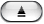Source: http://www.salto.at/Power20/Documentation/Power20-ReadMe/AA-VIC-20_BASIC.html Power20 Homepage: http://www.infinite-loop.at and http://www.salto.at - EMail: supportsaltoat © Roland Lieger, Goethegasse 39, A-2340 Mödling, Austria - Europe Last Changed: Feb. 29, 2008